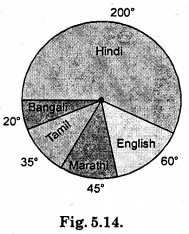# HBSE 8th Class Maths Solutions Chapter 5 Data Handling Ex 5.2

Haryana State Board HBSE 8th Class Maths Solutions Chapter 5 Data Handling Ex 5.2 Textbook Exercise Questions and Answers.

## Haryana Board 8th Class Maths Solutions Chapter 5 Data Handling Exercise 5.2

Question 1.
A survey was made to find the I type of music that a certain group of young people liked in a city. Adjoining pie chart shows the findings of this survey.
From this pie chart answer the following:
(i) If 20 people liked classical music, how many young people were surveyed ?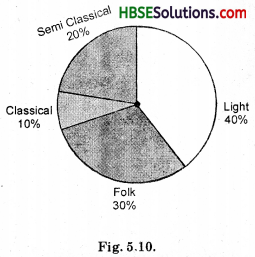(ii) Which type of music is liked by the maximum number of people ?
(iii) If a cassette company were to make 1000 CD’s, how many of each type would they make ?
Solution:
(i) No. of people liked classical music = 20.
Percentage of people liked classical music = 10%.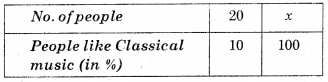This is in direct variation
$$\frac{20}{10}$$ = $$\frac{x}{100}$$
⇒ 10x = 20 × 100
⇒ x = $$\frac{2000}{10}$$ = 200 people.
Aliter : If 10% = 20 people 20
then 100% = $$\frac{20}{10 \%}$$ × 100%
= 200 people.

(ii) Light music.
(iii)No. of semi classical CD’s
= 20% of 1000 = $$\frac{20}{100}$$ × 1000 = 200
No. of classical music CD’s
= 10% of 1000 = $$\frac{10}{100}$$ × 1000 = 100
No. of Folk music CD’s
= $$\frac{30}{100}$$ × 1000 = 300
No. of light music CD’s 40
= $$\frac{40}{100}$$ × 1000 = 400.Question 2.
A group of 360 people were asked to vote for their favourite season from the three seasons rainy, winter and summer.
(i) Which season got the most votes ?
(ii) Find the central angle of each sector.
(iii) Draw a pie chart to show this information.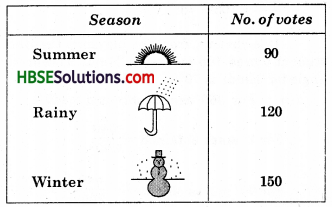Solution:
(i) Winter.
(ii) Central angle = $$\frac{Value of Item}{Total value}$$ 360°
∴ Central angle for Summer season
= $$\frac{90}{360}$$ × 360° = 90°
Central angle for Rainy season
= $$\frac{120}{360}$$ × 360° = 120°
and Central angle for Winter season
= $$\frac{150}{360}$$ × 360° = 150°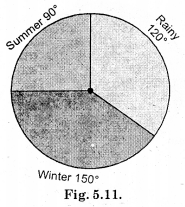Question 3.
Draw a pie chart showing the following information. The table shows the colours preferred by a group of people.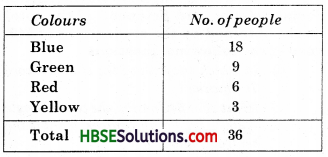Find the proportion of each sector. For example, Blue is $$\frac{18}{360}$$ = $$\frac{1}{2}$$; Green is $$\frac{9}{36}$$ = $$\frac{1}{4}$$ and so on. Use this to find the corresponding angles.
Solution: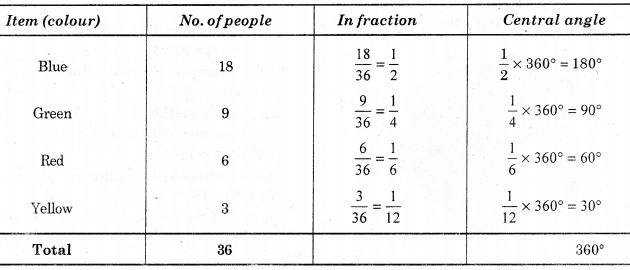Now, we make the pie chart as-shown below musing protractor, compasses and ruler.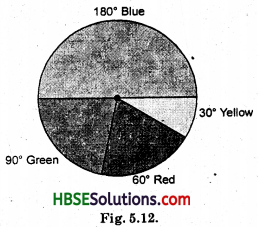Question 4.
The adjoining pie chart gives the marks scored in an examination by a student in Hindi, English, Mathematics, Social Science and Science. If the total marks obtained by the students were 540, answer the following questions :
(i) In which subject did the student score 105 marks ?
[Hint: For 540 marks, the central angle = 360°. So, for 105 marks, what is the central angle ?]
(ii) How many more marks were obtained by the student in Mathematics than in Hindi ?
(iii) Examine whether the sum of the marks obtained m Social Science and Mathematics is more than that in Science and Hindi.
[Hint: Just study the central angles.]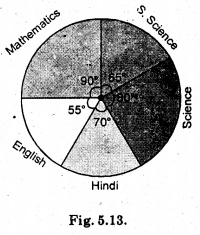Solution:
(i) For 540 marks, the central angle = 360°
So, for I mark, the central angle = $$\frac{360^{\circ}}{540}$$
So, for 105 marks, the central angle = $$\frac{360^{\circ}}{540}$$ × 108 = 70°
∴ It is obvious, from the pie chart that the student scored 105 marks, in ‘Hindi’ as central angle for ‘Hindi’ is 70°.

(ii) ∵ For 360° marks obtained = 540
∴ For 1° mark obtained = $$\frac{540}{360^{\circ}}$$
For 90° marks obtained = $$\frac{540}{360^{\circ}}$$ × 90°
∴ Marks obtained in Mathematics = 135
Marks obtained in Hindi = 105
Difference in marks = 135 – 105 = 30

(iii) Marks obtained in Social Science
= $$\frac{Total marks}{Total central angle}$$ × central angle of an Item
= $$\frac{540}{360^{\circ}}$$ × 65° = 97.5
Marks obtained in Science
= $$\frac{540}{360^{\circ}}$$ × 80° = 120
Marks obtained in (SST + Math)
= 97.5 + 135 = 232.5
Marks obtained in (Science + Hindi)
= 120 + 105 = 225
Alternative method:
Sum of central angles in (SST + Math)
= 65° + 90° = 155°
Sum of central angles in (Science + Hindi)
= 80 + 70 = 150°
So, sum of the marks obtained in SST and Mathematics is more than that in Science and Hindi.Question 5.
The number of students in a hostel, speaking different languages is given below. Display the data in a pie chart.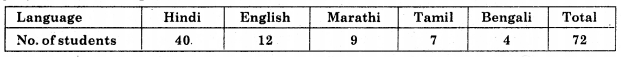Solution: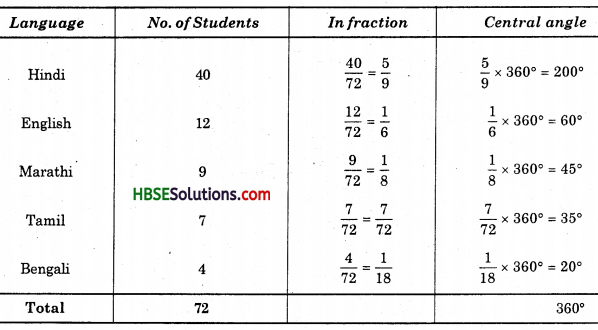Now, we make the pie chart as shown below